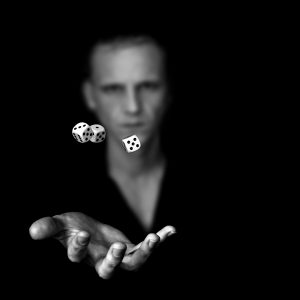Want to share your content on R-bloggers? click here if you have a blog, or here if you don't.This is the second exercise set on answering probability questions with simulation. Finishing the first exercise set is not a prerequisite. The difficulty level is about the same – thus if you are looking for a challenge aim at writing up faster more elegant algorithms.

As always, it pays off to read the instructions carefully and think about what the solution should be before starting to code. Often this helps you weed out irrelevant information that can otherwise make your algorithm unnecessarily complicated and slow.

Exercise 1
If you take cards numbered from 1-10 and shuffle them, and lay them down in order, what is the probability that at least one card matches its position. For example card 3 comes down third?

Exercise 2
Consider an election 3 candidates and 35 voters and who casts his ballot randomly for one of the candidates. What is the probability of a tie for the first position?

Exercise 3
If you were to randomly find a playing card on the floor every day, how many days would it take on average to find a full standard deck?

Exercise 4
Throw two dice. What is the probability the difference between them is 3, 4, or 5 instead of 0, 1, or 2?

Exercise 5
What is the expected number of distinct birthdays in a group of 400 people? Assume 365 days and that all are equally likely.

Exercise 6
What is the probability that a five-card hand in standard deck of cards has exactly three aces?

Learn more about probability functions in the online course Statistics with R – Advanced Level. In this course you will learn how to

• work with different binomial and logistic regression techniques,
• know how to compare regression models and choose the right fit,
• and much more.

Exercise 7
Randomly select three distinct integers a, b, c from the set {1, 2, 3, 4, 5, 6, 7}.
What is the probability that a + b > c?

Exercise 8
Given that a throw of three dice show three different faces what is the probability if the sum of all the dice is 8.

Exercise 9
Throwing a standard die until you get 6. What is the expected number of throws (including the throw giving 6) conditioned on the event that all throws gave even numbers.

Exercise 10
Choose two-digit integer at random, what is the probability that it is divisible by each of its digits. This is not exactly a simulation proplem – but the concept is similar make R do the hard work.

(Picture by Gil)